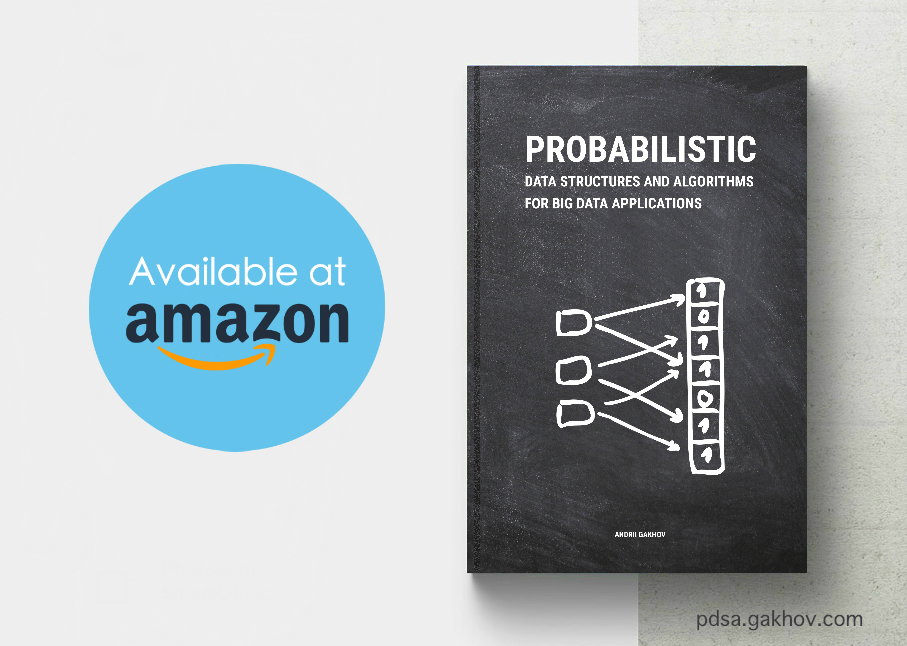Free cookie consent management tool by TermsFeed Policy Generator# Probabilistic Data Structures and Algorithms for Big Data Applications

## Hashing

We give a brief overview of popular hash functions and hash tables that are widely used in probabilistic data structures.

• Cryptographic hash functions
• Non-Cryptographic hash functions
• Hash tables

## Membership

We study the most well-known known probabilistic data structures that are used to answer approximate membership queries.

• Bloom filter
• Counting Bloom filter
• Quotient filter
• Cuckoo filter

## Cardinality

We learn data structures that allow to estimate the number of unique elements in a dataset.

• Linear Counting
• Probabilistic Counting
• LogLog and HyperLogLog

## Frequency

We discuss solutions for common problems in streaming applications, such as finding frequency of some element, filtering most frequent elements in the stream, detecting trending elements, etc.

• Majority algorithm
• Frequent algorithm
• Count Sketch
• Count–Min Sketch

## Rank

We study data structures to estimate quantiles and percentiles in a data stream using only one pass through the data.

• Random sampling
• q-digest
• t-digest

## Similarity

We learn how to effciently solve the nearest neighbor problem for large datasets using sub-linear in time solutions.

• Locality-Sensitive hashing
• MinHash
• SimHash

# Andrii Gakhov, Ph.D.

## Andrii Gakhov is a mathematician and software engineer holding a Ph.D.in mathematical modeling and numerical methods. He has been a teacher in the School of Computer Science at V. Karazin Kharkiv National University in Ukraine for a number of years and currently works as a software practitioner for ferret go GmbH, the leading community moderation, automation, and analytics company in Germany. His fields of interests include machine learning, stream mining, and data analysis.America
United States Amazon.com Visit
Mexico Amazon.com.mx Visit
Brazil Amazon.com.br Visit
Europe
United Kingdom Amazon.co.uk Visit
Germany BoD (Verlag) Visit
Thalia Visit
Amazon.de Visit
Austria BoD (Verlag) Visit
Amazon.de Visit
France Amazon.fr Visit
Spain Amazon.es Visit
Italy Amazon.it Visit
Netherlands BoD (Verlag) Visit
Asia
India Amazon.in Visit
Japan Amazon.co.jp Visit
Oceania
Australia Amazon.com.au Visit

## E-book editionExclusively at Amazon

America
United States Amazon.com Visit
Mexico Amazon.com.mx Visit
Brazil Amazon.com.br Visit
Europe
United Kingdom Amazon.co.uk Visit
Germany Amazon.de Visit
Austria Amazon.de Visit
France Amazon.fr Visit
Spain Amazon.es Visit
Italy Amazon.it Visit
Netherlands Amazon.nl Visit
Asia
India Amazon.in Visit
Japan Amazon.co.jp Visit
Oceania
Australia Amazon.com.au Visit

# Errata

## If you have found any error that is not in the list below, please contact us by email pdsa@gakhov.com. As soon as the error is confirmed and fixed, its description will appear in this section (details are omitted in mobile view due to space restrictions).

Edition Location Original text Corrected text
1st, 2019 p. vii footnote "What Is Big Data? https://www.ibm.com/software/data/bigdata/what-is-big-data.html" [removed]
1st, 2019 p. 31 "... by bitwise XOR, however ..." "... by bitwise AND, however ..."
1st, 2019 p. 38, Algorithm 2.8 $$\mathbf{QF}[i] \gets prev[i]$$ $$\mathbf{QF}[i] \gets prev$$
1st, 2019 p. 41, Example 2.9 ... $$16$$-bit fingerprints ... ... $$32$$-bit fingerprints ...
1st, 2019 p. 41, Example 2.9 $$h(x) = \text{MurmurHash3}(x) \bmod 16.$$ $$h(x) = \text{MurmurHash3}(x).$$
1st, 2019 p. 41, Example 2.9 $$p=13$$ $$p=29$$
1st, 2019 pp. 41, 43, Example 2.9 $$2^{13}$$ $$2^{29}$$
1st, 2019 pp. 42-45 ... remainder $$f_q$$ ... ... remainder $$f_r$$ ...
1st, 2019 p. 62, Example 3.1 "... of unique elements is 23 GB." "... of unique elements is 2.3 GB."
1st, 2019 p. 65, Algorithm 3.2 $$\mathbf{Add}(e, LINEARCOUNTER)$$ $$\mathbf{Add}(x, LINEARCOUNTER)$$
1st, 2019 p. 66, Example 3.3 $$V = \frac{9}{16} = 0.5625$$ $$V = \frac{7}{16} = 0.4375$$
1st, 2019 p. 66, Example 3.3 "... which is pretty close to ..." "... which is close but overestimates ...
1st, 2019 p. 66, Example 3.3 $$n \approx - 16 \cdot \ln{0.5625} \approx 9.206$$ $$n \approx - 16 \cdot \ln{0.4375} \approx 13.22$$
1st, 2019 p. 67 ... a fixed constant $$O(N)$$, where $$N$$ is the total number of elements, including duplicates. Thus, the algorithm has $$O(N)$$ time complexity. ... a fixed constant, and thus the algorithm has $$O(N)$$ time complexity, where $$N$$ is the total number of elements, including duplicates.
1st, 2019 p. 81, Formula 3.12 $$\hat n \approx \alpha_{m}\cdot m^{2} \cdot \left(\sum_{j=0}^{m-1}2^{-COUNTER[j]}\right)$$ $$\hat n \approx \alpha_{m}\cdot m^{2} \cdot \left(\sum_{j=0}^{m-1}2^{-COUNTER[j]}\right)^{-1}$$
1st, 2019 p. 96, Example 4.2 "... 500 billion ..." "... 500 million ..."
1st, 2019 p. 109, Example 4.5 $$s_1(4) = 1,\text{ }s_2(4) = 1,\text{ }s_2(4) = -1.$$ $$s_1(4) = 1,\text{ }s_2(4) = 1,\text{ }s_3(4) = -1.$$
1st, 2019 p. 135, Example 5.3 "... remaining empty buffer $$B_{2}$$." "... remaining empty buffer $$B_{4}$$."
1st, 2019 p. 159, Example 5.10 $$q(c_3) + \frac{1}{20} = \frac{6 + 11 + 1}{20} + \frac{1}{20} = 0.95,$$ $$q(c_3) - \frac{1}{20} = \frac{6 + 11 + 1}{20} - \frac{1}{20} = 0.85,$$
1st, 2019 p. 173, Example 6.8 $$c(d_1, d_4) = \frac{255 \cdot 0 + 0 \cdot 191 + 0 \cdot 255}{255 \cdot 255} = 0$$ $$c(d_1, d_4) = \frac{255 \cdot 0 + 0 \cdot 191 + 0 \cdot 255}{\sqrt{255^{2} + 0^2 + 0^2} \cdot \sqrt{0^{2} + 191^2 + 255^2}} = 0$$